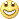# Stochastic Oscillator, the beginning - page 56154485

Try dss (it tends to show cycles on a 1 hour chart clearly)2047

Stochastic fast and stochastic slow (by apprentice)

Files:
sfk.mq4 3 kb
ssd.mq4 4 kb352

Hi MrTools

I try your indicator and I find it very interesting

Can you explain how you calculate that

If I don't write some mistake

To calculate the DSS, the formula is:

indic=exponentialaverage[r]((close-lowest[n](low))/(highest[n](high)-lowest[n](low)))

DSS=100*exponentialaverage[r]((indic-lowest[n](indic))/(highest[n](indic)-lowest[n](indic)))

So, do you replace close, low, and high by the RSX ?

Thanks a lot for your next answerZilliq

mrtools:
Hello Shtopr, Hope I understood added mtf.154485

zilliq:
Hi MrTools

I try your indicator and I find it very interesting

Can you explain how you calculate that

If I don't write some mistake

To calculate the DSS, the formula is:

indic=exponentialaverage[r]((close-lowest[n](low))/(highest[n](high)-lowest[n](low)))

DSS=100*exponentialaverage[r]((indic-lowest[n](indic))/(highest[n](indic)-lowest[n](indic)))

So, do you replace close, low, and high by the RSX ?

Thanks a lot for your next answerZilliq

Zilliq

It is replaced with a single value simply because at one bar rsx has just one value. The other parameters are there in order to be able to calculate the regular high/low ds stochastic (when it expects close,high and low, but even then you can use any combination of prices)352

Thanks Mladen for your anwserI'm not sure to understand

You mean that on the first line of code close, high and low are remplaced by RSX ? like that

indic=exponentialaverage[r]((rsx-lowest[n](rsx))/(highest[n](rsx)-lowest[n](rsx)))

and the DSS is the same as in my post ?

Zilliq154485

zilliq:
Thanks Mladen for your anwserI'm not sure to understand

You mean that on the first line of code close, high and low are remplaced by RSX ? like that

indic=exponentialaverage[r]((rsx-lowest[n](rsx))/(highest[n](rsx)-lowest[n](rsx)))

and the DSS is the same as in my post ?

Zilliq

Yes.

If you take a look at the iDss function and how it is called from the loop, you will see that it translates to exactly that352

ok thanks mladenhave a nice night

zilliq154485

DSS of momentum

Double smoothed stochastic of momentum. 3 different momentums used in calculation : momemntum of close, momentum of high and momentum of low. That way the double smoothed stochastic has almost "realistic" conditions for a perfect high-low stochastic calculation. That is why it can not be compared to a "simple" momentum : it is a combination of 3 momentums

_________________________

Updated version compatible with new metatrader builds posted here : https://www.mql5.com/en/forum/177239

Files:197

Double smoothed stochastic of momentum. 3 different momentums used in calculation : momemntum of close, momentum of high and momentum of low. That way the double smoothed stochastic has almost "realistic" conditions for a perfect high-low stochastic calculation. That is why it can not be compared to a "simple" momentum : it is a combination of 3 momentums

Dear Mladen,it looks good on any period. Thank you for your work.

Best regards,154485

DSS of MACD 3

Double smoothed stochastic of 3 macds : macd of close, macd of high and macd of low. Some experimenting with parameters advised

Files: